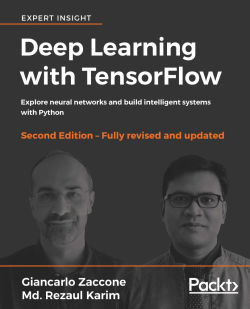### Deep Learning with TensorFlow - Second Edition4.5 (10 reviews total)
By Giancarlo Zaccone , Md. Rezaul Karim

• Constantly updated with 100+ new titles each month
• Breadth and depth in over 1,000+ technologies

Deep learning is a branch of machine learning algorithms based on learning multiple levels of abstraction. Neural networks, which are at the core of deep learning, are being used in predictive analytics, computer vision, natural language processing, time series forecasting, and to perform a myriad of other complex tasks.

This book is conceived for developers, data analysts, machine learning practitioners and deep learning enthusiasts who want to build powerful, robust, and accurate predictive models with the power of TensorFlow, combined with other open source Python libraries.

Throughout the book, you’ll learn how to develop deep learning applications for machine learning systems using Feedforward Neural Networks, Convolutional Neural Networks, Recurrent Neural Networks, Autoencoders, and Factorization Machines. Discover how to attain deep learning programming on GPU in a distributed way.

You'll come away with an in-depth knowledge of machine learning techniques and the skills to apply them to real-world projects.

Publication date:
March 2018
Publisher
Packt
Pages
484
ISBN
9781788831109

## Chapter 1. Getting Started with Deep Learning

This chapter explains some of the basic concepts of Machine Learning (ML) and Deep Learning (DL) that will be used in all the subsequent chapters. We will start with a brief introduction to ML. Then we will move to DL, which is a branch of ML based on a set of algorithms that attempt to model high-level abstractions in data.

We will briefly discuss some of the most well-known and widely used neural network architectures, before moving on to coding with TensorFlow in Chapter 2, A First Look at TensorFlow. In this chapter, we will look at various features of DL frameworks and libraries, such as the native language of the framework, multi-GPU support, and aspects of usability.

In a nutshell, the following topics will be covered:

• A soft introduction to ML

• Artificial neural networks

• ML versus DL

• DL neural network architectures

• Available DL frameworks

## A soft introduction to machine learning

ML is about using a set of statistical and mathematical algorithms to perform tasks such as concept learning, predictive modeling, clustering, and mining useful patterns. The ultimate goal is to improve the learning in such a way that it becomes automatic, so that no more human interactions are needed, or at least to reduce the level of human interaction as much as possible.

We now refer to a famous definition of ML by Tom M. Mitchell (Machine Learning, Tom Mitchell, McGraw Hill), where he explained what learning really means from a computer science perspective:

"A computer program is said to learn from experience E with respect to some class of tasks T and performance measure P, if its performance at tasks in T, as measured by P, improves with experience E."

Based on this definition, we can conclude that a computer program or machine can do the following:

• Learn from data and histories called training data

• Improve with experience

• Interactively enhance a model that can be used to predict outcomes of questions

Almost every machine-learning algorithm we use can be treated as an optimization problem. This is about finding parameters that minimize some objective function, such as a weighted sum of two terms such as a cost function and regularization (log-likelihood and log-prior, respectively, in statistics).

Typically, an objective function has two components: a regularizer, which controls the complexity of the model, and the loss, which measures the error of the model on the training data (we’ll look into the details).

On the other hand, the regularization parameter defines the trade-off between the two goals of minimizing the loss of the training error and of minimizing the model's complexity in an effort to avoid overfitting. Now if both of these components are convex, then their sum is also convex; else it is nonconvex.

### Note

In machine learning, overfitting is when the predictor model fits perfectly on the training examples, but does badly on the test examples. This often happens when the model is too complex and trivially fits the data (too many parameters), or when there is not enough data to accurately estimate the parameters. When the ratio of model complexity to training set size is too high, overfitting will typically occur.

More elaborately, while using an ML algorithm, our goal is to obtain the hyperparameters of a function that returns the minimum error when making predictions. The error loss function has a typically U-shaped curve, when visualized on a two-dimensional plane, and there exists a point, which gives the minimum error.

Therefore, using a convex optimization technique, we can minimize the function until it converges toward the minimum error (that is, it tries to reach the middle region of the curve), which represents the minimum error. Now that a problem is convex, it is usually easier to analyze the asymptotic behavior of the algorithm that shows how fast it converges as the model observes more and more training data.

The challenge of ML is to allow a computer to learn how to automatically recognize complex patterns and make decisions as intelligently as possible. The entire learning process requires a dataset, as follows:

• Training set: This is the knowledge base used to fit the parameters of the machine-learning algorithm. During this phase, we would use the training set to find the optimal weights, with the back-prop rule, and all the parameters to set before the learning process begins (hyperparameters).

• Validation set: This is a set of examples used to tune the parameters of an ML model. For example, we would use the validation set to find the optimal number of hidden units, or determine a stopping point for the back-propagation algorithm. Some ML practitioners refer to it as development set or dev set.

• Test set: This is used for evaluating the performance of the model on unseen data, which is called model inferencing. After assessing the final model on the test set, we don't have to tune the model any further.

Learning theory uses mathematical tools that derive from probability theory and information theory. Three learning paradigms will be briefly discussed:

• Supervised learning

• Unsupervised learning

• Reinforcement learning

The following diagram summarizes the three types of learning, along with the problems they address: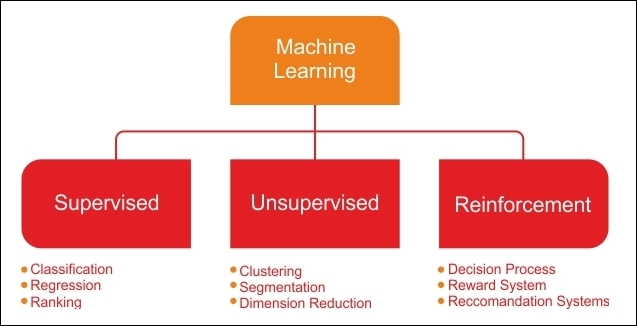Figure 1: Types of learning and related problems.

### Supervised learning

Supervised learning is the simplest and most well-known automatic learning task. It is based on a number of pre-defined examples, in which the category where each of the inputs should belong is already known. In this case, the crucial issue is the problem of generalization. After the analysis of a typical small sample of examples, the system should produce a model that should work well for all possible inputs.

The following figure shows a typical workflow of supervised learning. An actor (for example, an ML practitioner, data scientist, data engineer, or ML engineer) performs ETL (Extraction, Transformation, and Load) and necessary feature engineering (including feature extraction, selection) to get the appropriate data, with features and labels.

Then he does the following:

• Splits the data into the training, development, and test set

• Uses the training set to train an ML model

• Uses the validation set for validating the training against the overfitting problem, and regularization

• Evaluates the model's performance on the test set (that is, unseen data)

• Finally, he deploys the best model into a production-ready environment

In the overall lifecycle, there might be many actors involved (for example, data engineer, data scientist, or ML engineer) to perform each step independently or collaboratively: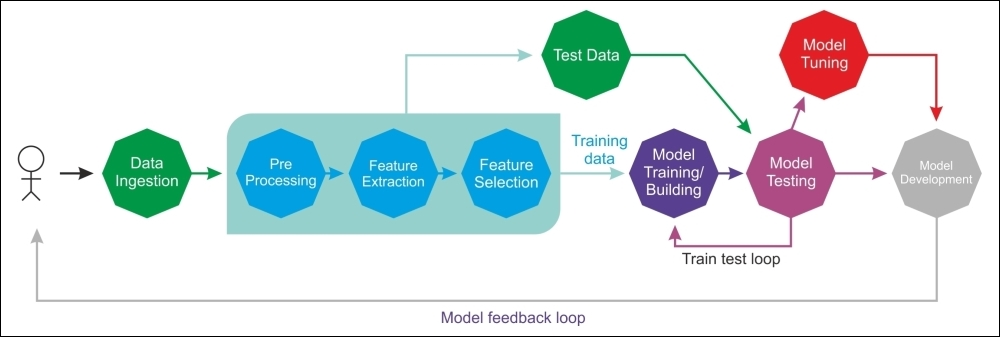Figure 2: Supervised learning in action.

In supervised ML, the set consists of labeled data, that is, objects and their associated values for regression. This set of labeled examples, therefore, constitutes the training set. Most supervised learning algorithms share one characteristic: the training is performed by the minimization of a particular loss or cost function, representing the output error provided by the system, with respect to the desired output.

The supervised learning context includes classification and regression tasks: classification is used to predict which class a data point is a part of (discrete value) while regression is used to predict continuous values: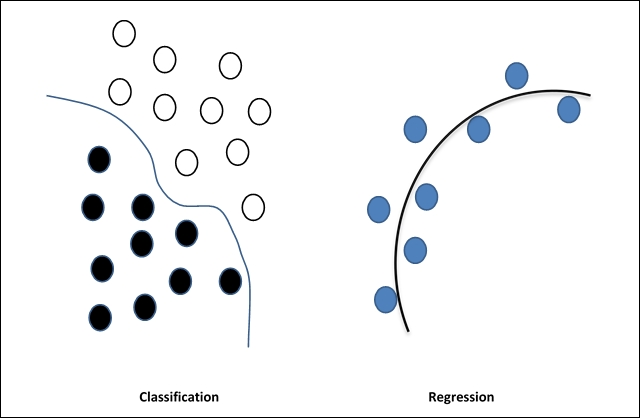Figure 3: Classification and regression

In other words, the classification task predicts the label of the class attribute, while the regression task makes a numeric prediction of the class attribute.

### Unbalanced data

In the context of supervised learning, unbalanced data refers to classification problems where we have unequal instances for different classes. For example, if we have a classification task for only two classes, balanced data would mean 50% preclassified examples for each of the classes.

If the input dataset is a little unbalanced (for example, 60% for one class and 40% for the other class) the learning process will be required to randomly split the input dataset into three sets, with 50% for the training set, 20% for the validation set, and the remaining 30% for the testing set.

### Unsupervised learning

In unsupervised learning, an input set is supplied to the system during the training phase. In contrast with supervised learning, the input objects are not labeled with their class. This type of learning is important because, in the human brain, it is probably far more common than supervised learning.

For the classification, we assume that we are given a training dataset of correctly labeled data. Unfortunately, we do not always have that luxury when we collect data in the real world. The only object in the domain of learning models, in this case, is the observed data input, which is often assumed to be independent samples of an unknown underlying probability distribution.

For example, suppose that you have a large collection of non-pirated and totally legal MP3s in a crowded and massive folder on your hard drive. How could you possibly group together songs without direct access to their metadata? One possible approach could be a mixture of various ML techniques, but clustering is often at the heart of the solution.

Now, what if you could build a clustering predictive model that could automatically group together similar songs, and organize them into your favorite categories such as "country", "rap" and "rock"? The MP3 would be added to the respective playlist in an unsupervised way. In short, unsupervised learning algorithms are commonly used in clustering problems: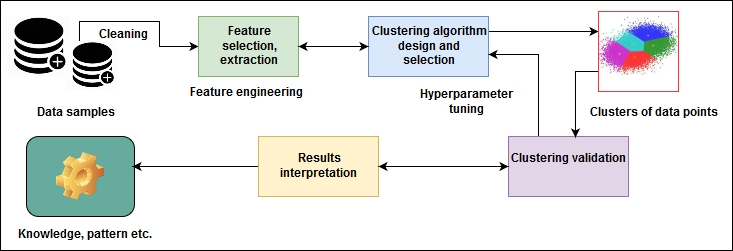Figure 4: Clustering techniques: an example of unsupervised learning

See the preceding diagram to get an idea of a clustering technique being applied to solve this kind of problem. Although the data points are not labeled, we can still do the necessary feature engineering, and group a set of objects in such a way that objects in the same group (called a cluster) are more similar (in some sense) to each other, than to those in other groups (clusters).

This is not easy for a human, because a standard approach is to define a similarity measure between two objects and then look for any cluster of objects that are more similar to each other than they are to the objects in the other clusters. Once we do the clustering, the validation of data points (that is, MP3 files) is completed and we know the pattern of the data (that is, what type of MP3 files fall in to which group).

### Reinforcement learning

Reinforcement learning is an artificial intelligence approach that focuses on the learning of the system through its interactions with the environment. With reinforcement learning, the system adapts its parameters based on feedback received from the environment, which then provides feedback on the decisions made. The following diagram shows a person making decisions in order to arrive at their destination. Suppose that, on your drive from home to work, you always choose the same route. However, one day your curiosity takes over and you decide to try a different route, in the hope of finding a shorter commute. This dilemma of trying out new routes, or sticking to the best-known route, is an example of exploration versus exploitation: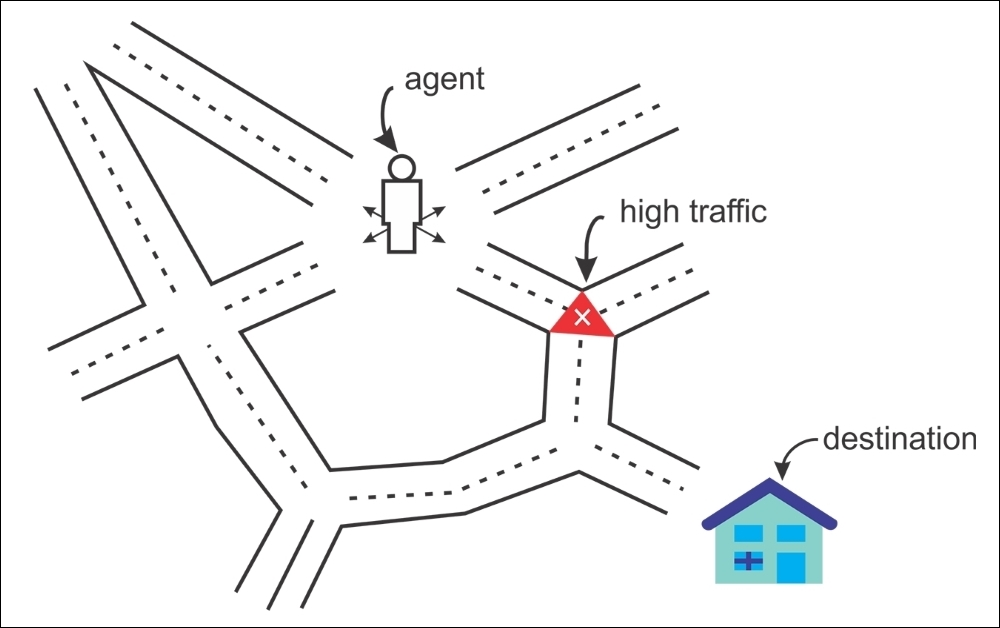Figure 5: An agent always tries to reach the destination.

Another example is a system that models a chess player, that uses the result of its preceding moves to improve its performance. This is a system that learns with reinforcement.

Current research on reinforcement learning is highly interdisciplinary, including researchers specializing in genetic algorithms, neural networks, psychology, and control engineering.

### What is deep learning?

Simple ML methods that were used in the normal size data analysis are not effective anymore, and should be substituted for more robust ML methods. Although classical ML techniques allow researchers to identify groups, or clusters, of related variables, the accuracy and effectiveness of these methods diminishes with large and high-dimensional datasets.

Therefore, here comes DL, which is one of the most important developments in artificial intelligence in the last few years. DL is a branch of ML based on a set of algorithms that attempt to model high-level abstractions in data.

The development of DL occurred in parallel with the study of artificial intelligence, and especially with the study of neural networks. It was mainly in the 1980s that this area grew, thanks largely to Geoff Hinton and the ML specialists who collaborated with him. At that time, computer technology was not sufficiently advanced to allow a real improvement in this direction, so we had to wait for a greater availability of data and vastly improved computing power to see significant developments.

In short, DL algorithms are a set of Artificial Neural Networks (ANNs), which we will explore later, that can make better representations of large-scale datasets, in order to build models that learn these representations extensively. In this regard, Ian Goodfellow and others defined DL as follows:

"Deep learning is a particular kind of machine learning that achieves great power and flexibility by learning to represent the world as a nested hierarchy of concepts, with each concept defined in relation to simpler concepts, and more abstract representations computed in terms of less abstract ones".

Let's give an example. Suppose we want to develop a predictive analytics model, such as an animal recognizer, where our system has to resolve two problems:

1. Classify if an image represents a cat or a dog

2. Cluster dog and cat images

If we solve the first problem using a typical ML method, we must define the facial features (ears, eyes, whiskers, and so on), and write a method to identify which features (typically non-linear) are more important when classifying a particular animal.

However, at the same time, we cannot address the second problem, because classical ML algorithms for clustering images (such as K-means) cannot handle non-linear features.

DL algorithms will take these two problems one step further and the most important features will be extracted automatically, after determining which features are the most important for classification or clustering. In contrast, using a classic ML algorithm, we would have to manually provide the features.

In summary, the DL workflow would be as follows:

• A DL algorithm would first identify the edges that are most relevant when clustering cats or dogs

• It would then build on this hierarchically to find the various combinations of shapes and edges

• After consecutive hierarchical identification of complex concepts and features, it decides which of these features can be used to classify the animal, then takes out the label column and performs unsupervised training using an autoencoder, before doing the clustering.

Up to this point, we have seen that DL systems are able to recognize what an image represents. A computer does not see an image as we see it because it only knows the position of each pixel and its color. Using DL techniques, the image is divided into various layers of analysis. At a lower level, the software analyzes, for example, a grid of a few pixels, with the task of detecting a type of color or various nuances. If it finds something, it informs the next level, which at this point verifies whether that given color belongs to a larger form, such as a line.

The process continues to the upper levels until you understand what is shown in the image. Software capable of doing these things is now widespread and is found in systems for recognizing faces or searching for an image on Google, for example. In many cases, these are hybrid systems, that work with more traditional IT solutions, that are mixed with generation artificial intelligence.

The following diagram shows what we have discussed in the case of an image classification system. Each block gradually extracts the features of the input image and goes on to process data from the previous blocks, that have already been processed, extracting increasingly abstract features of the image, and thus building the hierarchical representation of data that comes with a DL-based system.

More precisely, it builds the layers as follows:

• Layer 1: The system starts identifying the dark and light pixels

• Layer 2: The system identifies edges and shapes

• Layer 3: The system learns more complex shapes and objects

• Layer 4: The system learns which objects define a human face

This is shown in the following diagram: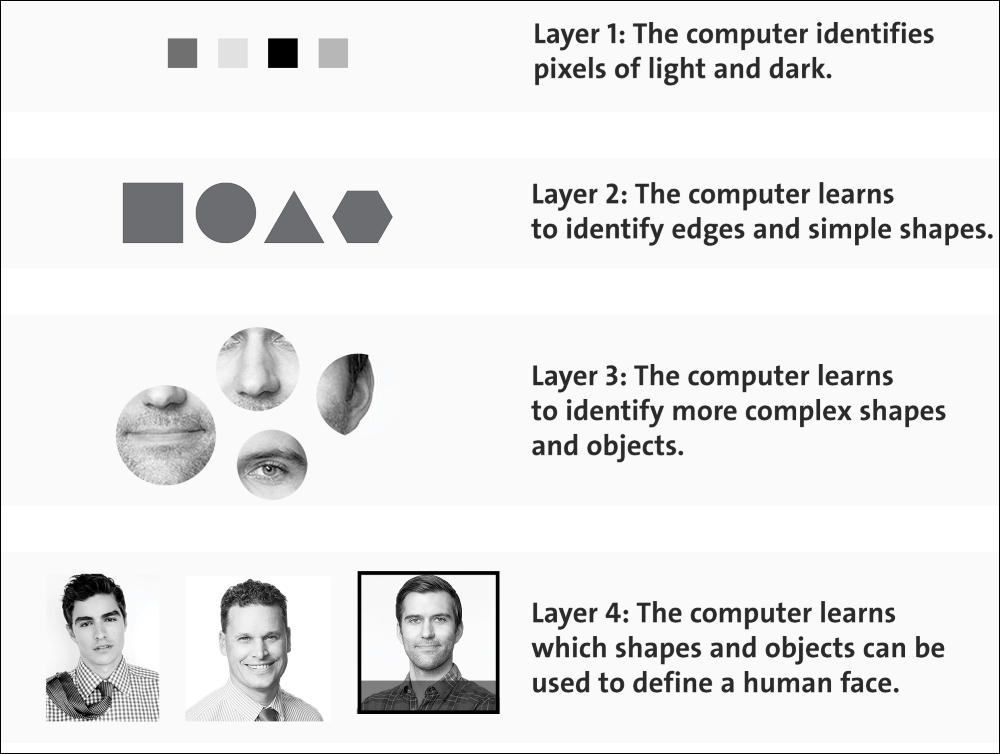Figure 6: A DL system at work on a facial classification problem.

In the previous section, we saw that using a linear ML algorithm, we typically handle only a few hyperparameters.

However, when neural networks come in the party, things become too complex. In each layer, there are so many hyperparameters, and the cost function always becomes nonconvex.

Another reason is that the activations functions used in the hidden layers are nonlinear, so the cost is nonconvex. We’ll discuss this phenomenon in more detail in the later chapters.

## Artificial neural networks

ANNs take advantage of the concept of DL. They are an abstract representation of the human nervous system, which contains a collection of neurons that communicate with each other through connections called axons.

Warren McCulloch and Walter Pitts proposed the first artificial neuron model in 1943 in terms of a computational model of nervous activity. This model was followed by another proposed by John von Neumann, Marvin Minsky, Frank Rosenblatt (the so-called perceptron), and many others.

### The biological neurons

Look at the brain's architecture for inspiration. Neurons in the brain are called biological neurons. They are unusual–looking cells, mostly found in animal brains, consisting of cortexes. The cortex itself is composed of a cell body, containing the nucleus and most of the cell's complex components. There are many branching extensions called dendrites, plus one very long extension called the axon.

Near its extremity, the axon splits off into many branches called telodendria and at the top of these branches are minuscule structures called synaptic terminals (or simply synapses), which connect to the dendrites of other neurons. Biological neurons receive short electrical impulses called signals from other neurons, and in response, they fire their own signals: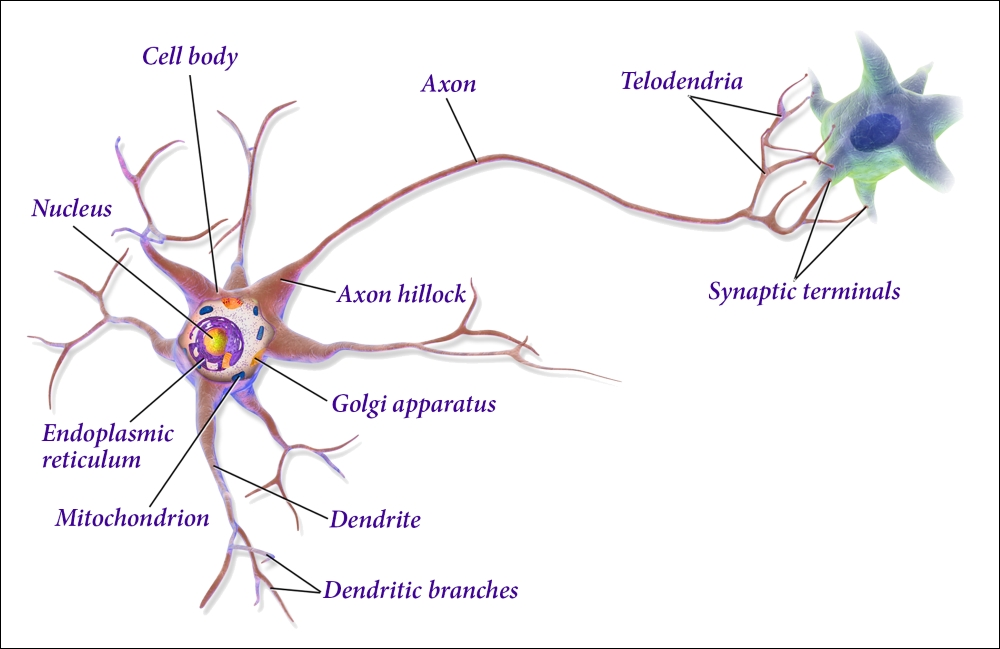Figure 7: Working principles of biological neurons.

In biology, a neuron is composed of the following:

• A cell body or soma

• One or more dendrites, whose responsibility it is to receive signals from other neurons

• An axon, which in turn conveys the signals generated by the same neuron to the other connected neurons

The neuron's activity alternates between sending a signal (active state) and rest/receiving signals from other neurons (inactive state). The transition from one phase to another is caused by the external stimuli, represented by signals that are picked up by the dendrites. Each signal has an excitatory or inhibitory effect, conceptually represented by a weight associated with the stimulus.

A neuron in idle state accumulates all the signals it has received until it reaches a certain activation threshold.

### The artificial neuron

Based on the concept of biological neurons, the term and the idea of artificial neurons arose, and they have been used to build intelligent machines for DL-based predictive analytics. This is the key idea that inspired ANNs. Similarly to biological neurons, the artificial neuron consists of the following:

• One or more incoming connections, with the task of collecting numerical signals from other neurons: each connection is assigned a weight that will be used to consider each signal sent

• One or more output connections that carry the signal to the other neurons

• An activation function, which determines the numerical value of the output signal, based on the signals received from the input connections with other neurons, and suitably collected from the weights associated with each received signal, and the activation threshold of the neuron itself: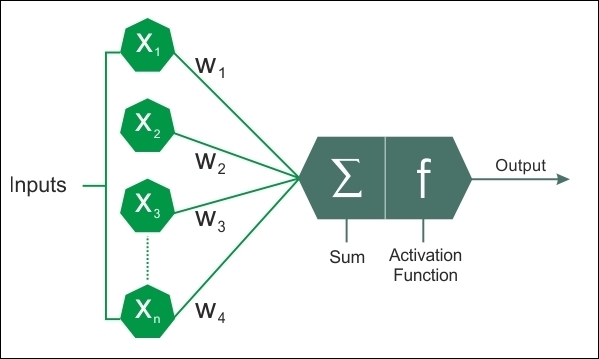Figure 8: Artificial neuron model.

The output, that is, the signal that the neuron transmits, is calculated by applying the activation function, also called the transfer function, to the weighted sum of the inputs. These functions have a dynamic range between -1 and 1, or between 0 and 1. Many activation functions differ in terms of complexity and output. Here, we briefly present the three simplest forms:

• Step function: Once we fix the threshold value x (for example, x = 10), the function will return zero, or one if the mathematical sum of the inputs is at, above, or below the threshold value.

• Linear combination: Instead of managing a threshold value, the weighted sum of the input values is subtracted from a default value. We will have a binary outcome that will be expressed by a positive (+b) or negative (-b) output of the subtraction.

• Sigmoid: This produces a sigmoid curve, which is a curve with an S trend. Often, the sigmoid function refers to a special case of the logistic function.

From the simplest forms used in the prototyping of the first artificial neurons, we move to more complex forms that allow greater characterization of the functioning of the neuron:

• Hyperbolic tangent function

• Conic section function

• Softmax function: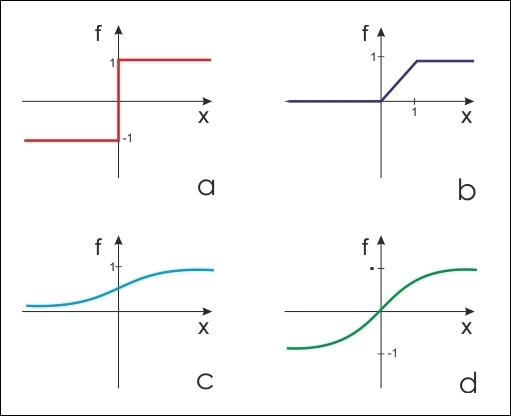Figure 9: The most commonly used artificial neuron model transfer functions. a. step function. b. linear function c. computed sigmoid function with values between 0 and 1. d. sigmoid function with computed values between -1 and 1.

Choosing proper activation functions (also weights initialization) is key to making a network perform at its best and to obtain good training. These topics are under a lot of research, and studies indicate marginal differences in terms of output quality if the training phase is carried out properly.

### Note

There is no rule of thumb in the field of neural networks. It all depends on your data and in what form you want the data to be transformed, after passing through the activation function. If you want to choose a particular activation function, you need to study the graph of the function to see how the result changes with respect to the values given to it.

## How does an ANN learn?

The learning process of a neural network is configured as an iterative process of the optimization of the weights and is therefore of the supervised type. The weights are modified because of the network's performance on a set of examples belonging to the training set, that is, the set where you know the classes that the examples belong to.

The aim is to minimize the loss function, which indicates the degree to which the behavior of the network deviates from the desired behavior. The performance of the network is then verified on a testing set consisting of objects (for example, images in an image classification problem) other than those in the training set.

### ANNs and the backpropagation algorithm

A commonly used supervised learning algorithm is the backpropagation algorithm. The basic steps of the training procedure are as follows:

1. Initialize the net with random weights

2. For all training cases, follow these steps:

• Forward pass: Calculates the network's error, that is, the difference between the desired output and the actual output

• Backward pass: For all layers, starting with the output layer back to input layer:

i: Shows the network layer's output with the correct input (error function).

ii: Adapts the weights in the current layer to minimize the error function. This is backpropagation's optimization step.

The training process ends when the error on the validation set begins to increase because this could mark the beginning of a phase overfitting, that is, the phase in which the network tends to interpolate the training data at the expense of generalizability.

### Weight optimization

The availability of efficient algorithms to optimize weights, therefore, constitutes an essential tool for the construction of neural networks. The problem can be solved with an iterative numerical technique called Gradient Descent (GD). This technique works according to the following algorithm:

1. Randomly choose initial values for the parameters of the model

2. Compute the gradient G of the error function with respect to each parameter of the model

3. Change the model's parameters so that they move in the direction of decreasing the error, that is, in the direction of -G

4. Repeat steps 2 and 3 until the value of G approaches zero

The gradient (G) of the error function E provides the direction in which the error function with the current values has the steeper slope; so to decrease E, we have to make some small steps in the opposite direction, -G.

By repeating this operation several times in an iterative manner, we move down towards the minimum of E, to reach a point where G = 0, in such a way that no further progress is possible: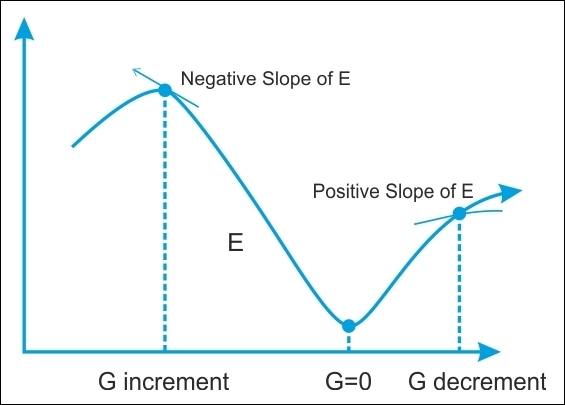Figure 10: Searching for the minimum for the error function E. We move in the direction in which the gradient G of the function E is minimal.

In GD optimization, we compute the cost gradient based on the complete training set, so we sometimes also call it batch GD. In the case of very large datasets, using GD can be quite costly, since we are only taking a single step for one pass over the training set. The larger the training set, the more slowly our algorithm updates the weights, and the longer it may take until it converges at the global cost minimum.

The fastest method of gradient descent is Stochastic Gradient Descent (SGD), and for this reason, it is widely used in deep neural networks. In SGD, we use only one training sample from the training set to do the update for a parameter in a particular iteration.

Here, the term stochastic comes from the fact that the gradient based on a single training sample is a stochastic approximation of the true cost gradient. Due to its stochastic nature, the path towards the global cost minimum is not direct, as in GD, but may zigzag if we are visualizing the cost surface in a 2D space: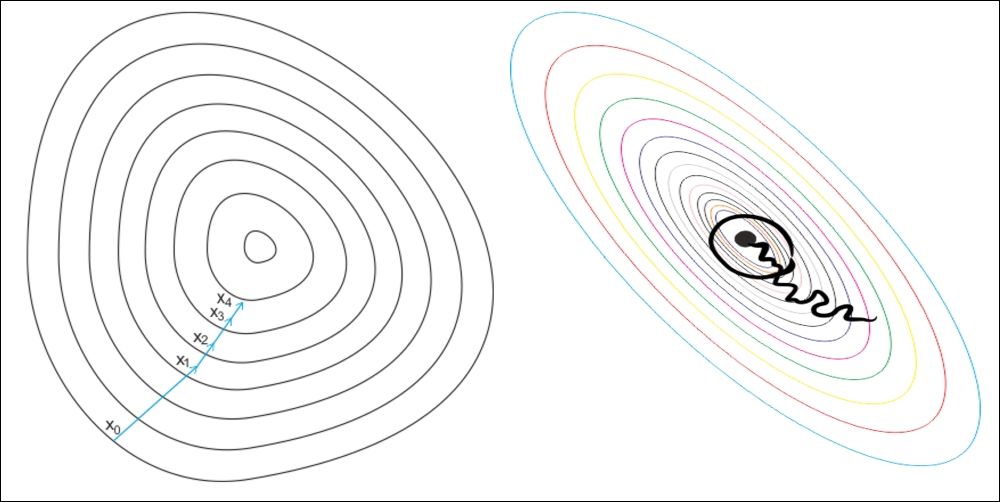Figure 11: GD versus SGD: the gradient descent (left figure) ensures that each update in the weights is done in the right direction: the direction that minimizes the cost function. With the growth in the dataset's size, and more complex computations in each step, SGD (right figure) is preferred in these cases. Here, updates to the weights are done as each sample is processed and, as such, subsequent calculations already use improved weights. Nonetheless, this very reason leads to some misdirection in minimizing the error function.

## Neural network architectures

The way that we connect the nodes and the number of layers present (that is, the levels of nodes between input and output, and the number of neurons per layer), defines the architecture of a neural network.

There are various types of architectures in neural networks. We can categorize DL architectures into four groups: Deep Neural Networks (DNNs), Convolutional Neural Networks (CNNs), Recurrent Neural Networks (RNNs), and Emergent Architectures (EAs). The following sections of this chapter will offer a brief introduction to these architectures. A more detailed analysis, with examples of applications, will be the subject of the following chapters of this book.

### Deep Neural Networks (DNNs)

DNNs are ANNs which are strongly oriented to DL. Where normal procedures of analysis are inapplicable, due to the complexity of the data to be processed, such networks are therefore an excellent modeling tool. DNNs are neural networks that are very similar to those we have discussed, but they must implement a more complex model (a greater number of neurons, hidden layers, and connections), although they follow the learning principles that apply to all ML problems (such as supervised learning). The computation in each layer transforms the representations in the layer below into slightly more abstract representations.

We will use the term DNN to refer specifically to Multilayer Perceptron (MLP), Stacked Auto-Encoder (SAE), and Deep Belief Networks (DBNs). SAEs and DBNs use AutoEncoders (AEs) and RBMs as building blocks of the architectures. The main difference between them and MLP is that training is executed in two phases: unsupervised pre-training and supervised fine-tuning: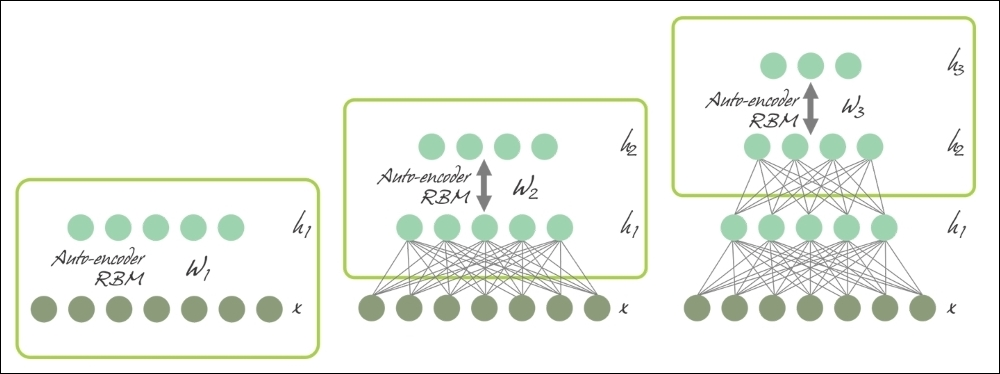Figure 12: SAE and DBN using AE and RBM respectively.

In unsupervised pre-training, shown in the preceding diagram, the layers are stacked sequentially and trained in a layer-wise manner, like an AE or RBM using unlabeled data. Afterwards, in supervised fine-tuning, an output classifier layer is stacked, and the complete neural network is optimized, by retraining with labeled data.

In this chapter, we will not discuss SAEs (see more details in Chapter 5, Optimizing TensorFlow Autoencoders), but will stick to MLPs and DBNs and use these two DNN architectures. We will see how to develop predictive models to deal with high-dimensional datasets.

#### Multilayer perceptron

In multilayer networks, you can identify the artificial neurons of the layers, so that each neuron is connected to all those in the next layer, ensuring that:

• There are no connections between neurons belonging to the same layer

• There are no connections between neurons belonging to non-adjacent layers

• The number of layers and neurons per layer depends on the problem to be solved

The input and output layers define inputs and outputs, and there are hidden layers, whose complexity realizes different behaviors of the network. Finally, the connections between neurons are represented by as many matrices as the pairs of adjacent layers.

Each array contains the weights of the connections between the pairs of nodes of two adjacent layers. The feedforward networks are networks with no loops within the layers.

We will describe feedforward networks in more detail in Chapter 3, Feed-Forward Neural Networks with TensorFlow: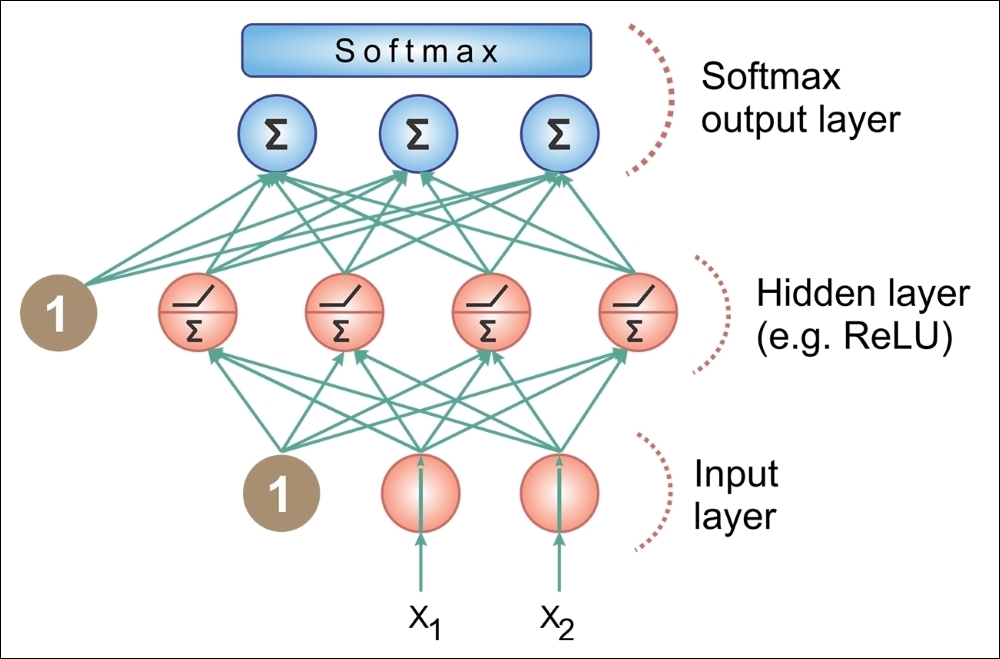Figure 13: MLP architecture

#### Deep Belief Networks (DBNs)

To overcome the overfitting problem in MLP, we set up a DBN, do unsupervised pre-training to get a decent set of feature representations for the inputs, then fine-tune the training set to get actual predictions from the network. While the weights of an MLP are initialized randomly, a DBN uses a greedy layer-by-layer pre-training algorithm to initialize the network weights through probabilistic generative models. The models are composed of a visible layer and multiple layers of stochastic and latent variables, which are called hidden units or feature detectors.

DBNs are Deep Generative Models, which are neural network models that can replicate the data distribution that you provide. This allows you to generate "fake-but-realistic" data points from real data points.

DBNs are composed of a visible layer and multiple layers of stochastic, latent variables, which are called hidden units or feature detectors. The top two layers have undirected, symmetric connections between them and form an associative memory, whereas lower layers receive top-down, directed connections from the preceding layer. The building blocks of DBNs are Restricted Boltzmann Machines (RBMs). As you can see in the following figure, several RBMs are stacked one after another to form DBNs: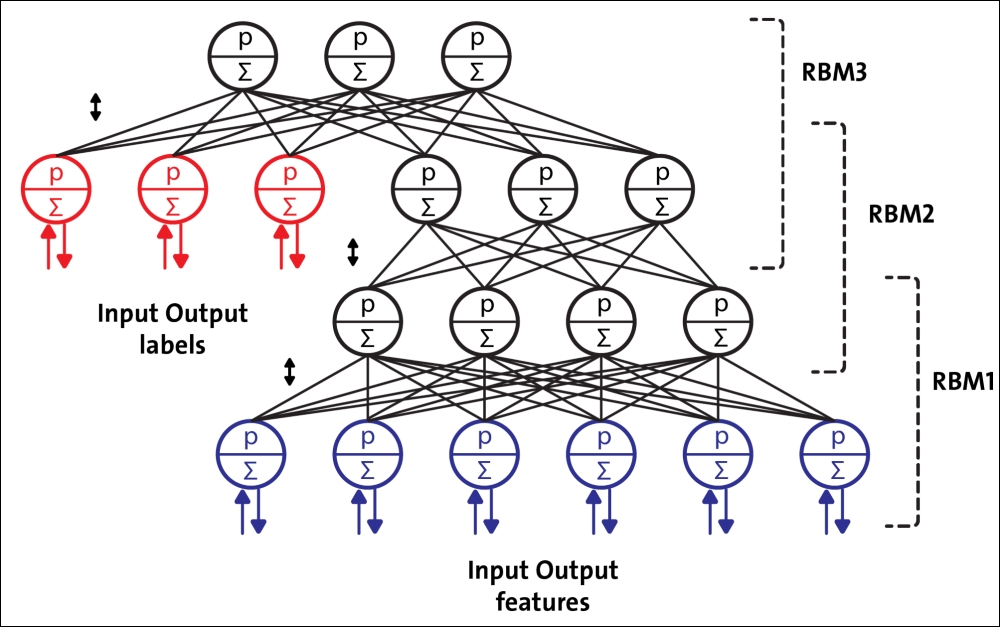Figure 14: A DBN configured for semi-supervised learning

A single RBM consists of two layers. The first layer is composed of visible neurons, and the second layer consists of hidden neurons. The following figure shows the structure of a simple RBM. Visible units accept inputs, and hidden units are nonlinear feature detectors. Each visible neuron is connected to all the hidden neurons, but there is no internal connection among neurons in the same layer.

An RBM consists of a visible layer node and a hidden layer node, but without visible-visible and hidden-hidden connections, hence the term restricted. They allow more efficient network training that can be supervised or unsupervised. This type of neural network is able to represent a large number of features of the inputs, then hidden nodes can represent up to 2n features. The network can be trained to respond to a single question (for example, yes or no to the question: Is it a cat?) until it can respond (again in binary terms) to a total of 2n questions (Is it a cat?, It is Siamese?, Is it white?).

The architecture of the RBM is as follows, with neurons arranged according to a symmetrical bipartite graph: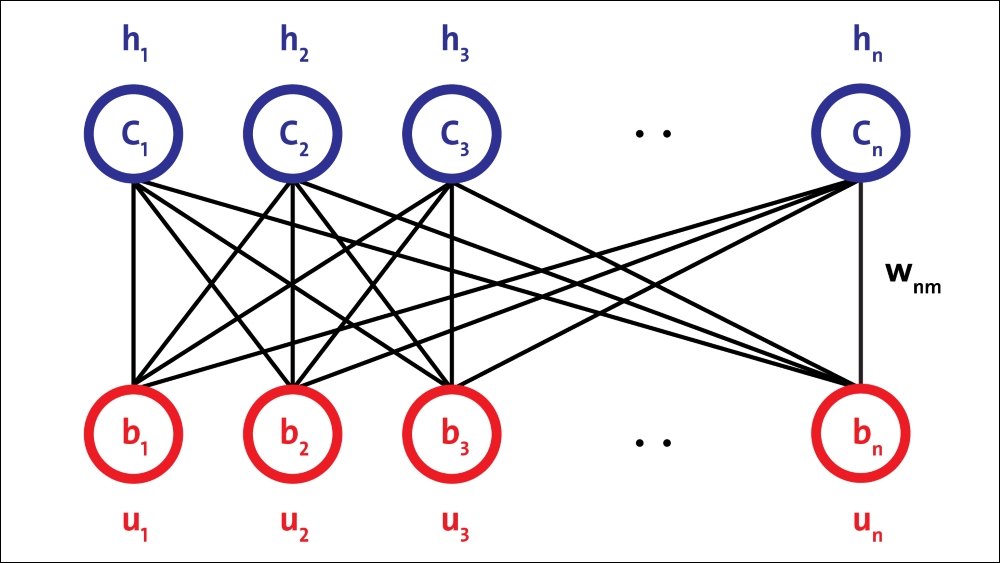Figure 15: RBM architecture.

A single hidden layer RBM cannot extract all the features from the input data, due to its inability to model the relationship between variables. Hence, multiple layers of RBMs are used one after another to extract nonlinear features. In DBNs, an RBM is trained first with input data, and the hidden layer represents the features learned using a greedy learning approach. These learned features of the first RBM, that is, a hidden layer of the first RBM, are used as the input to the second RBM, as another layer in the DBN.

Similarly, the learned features of the second layer are used as input for another layer. This way, DBNs can extract deep and nonlinear features from input data. The hidden layer of the last RBM represents the learned features of the whole network.

### Convolutional Neural Networks (CNNs)

CNNs have been specifically designed for image recognition. Each image used in learning is divided into compact topological portions, each of which will be processed by filters to search for particular patterns. Formally, each image is represented as a three-dimensional matrix of pixels (width, height, and color), and every sub-portion can be placed on convolution with the filter set. In other words, scrolling each filter along the image computes the inner product of the same filter and input.

This procedure produces a set of feature maps (activation maps) for the various filters. Superimposing the various feature maps onto the same portion of the image, we get an output volume. This type of layer is called the convolutional layer. The following diagram is a schematic of the architecture of a CNN: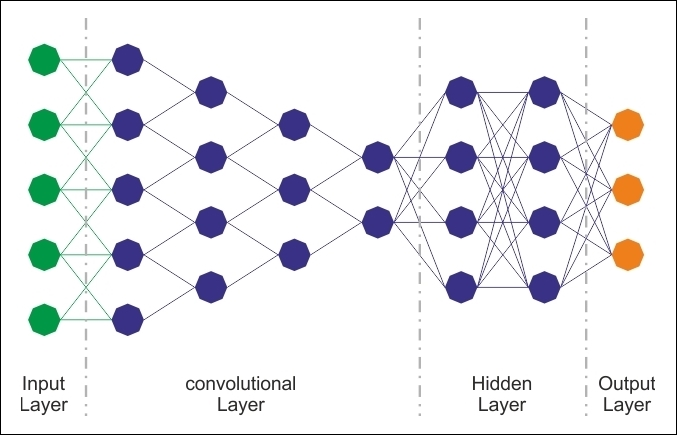Figure 16: CNN architecture.

Although regular DNNs work fine for small images (for example, MNIST and CIFAR-10), they break down with larger images because of the huge number of parameters required. For example, a 100×100 image has 10,000 pixels, and if the first layer has just 1,000 neurons (which already severely restricts the amount of information transmitted to the next layer), this means 10 million connections. In addition, that is just for the first layer.

CNNs solve this problem using partially connected layers. Because consecutive layers are only partially connected and because it heavily reuses its weights, a CNN has far fewer parameters than a fully connected DNN, which makes it much faster to train. This reduces the risk of overfitting and requires much less training data. Moreover, when a CNN has learned a kernel that can detect a particular feature, it can detect that feature anywhere on the image. In contrast, when a DNN learns a feature in one location, it can detect it only in that particular location. Since images typically have very repetitive features, CNNs are able to generalize much better than DNNs on image processing tasks such as classification and use fewer training examples.

Importantly, the DNN has no prior knowledge of how the pixels are organized; it does not know that nearby pixels are close. A CNN's architecture embeds this prior knowledge. Lower layers typically identify features in small areas of the images, while higher layers combine the lower-level features into larger features. This works well with most natural images, giving CNNs a decisive head-start over DNNs: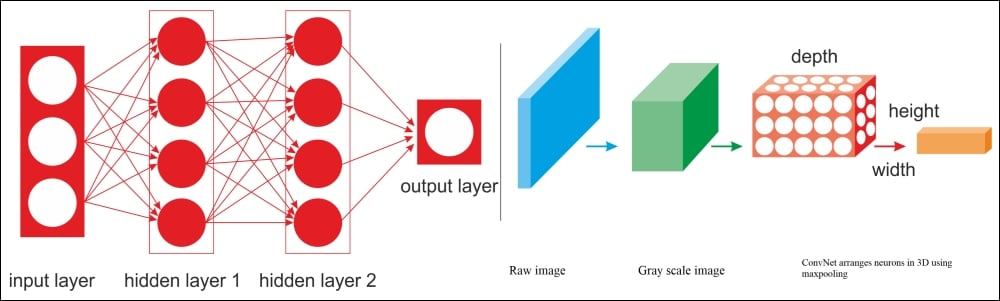Figure 17: A regular DNN versus a CNN.

For example, in the preceding diagram, on the left, you can see a regular three-layer neural network. On the right, a CNN arranges its neurons in three dimensions (width, height, and depth), as visualized in one of the layers. Every layer of a CNN transforms the 3D input volume to a 3D output volume of neuron activations. The red input layer holds the image, so its width and height would be the dimensions of the image, and the depth would be three (red, green and blue channels).

Therefore, all the multilayer neural networks we looked at had layers composed of a long line of neurons, and we had to flatten input images or data to 1D before feeding them to the neural network. However, what happens when you try to feed them a 2D image directly? The answer is that in a CNN, each layer is represented in 2D, which makes it easier to match neurons with their corresponding inputs. We will see examples of this in upcoming sections.

### AutoEncoders

An AE is a network with three or more layers, where the input layer and the output have the same number of neurons, and those intermediate (hidden layers) have a lower number of neurons. The network is trained to simply reproduce in the output, for each piece of input data, the same pattern of activity in the input.

AEs are ANNs capable of learning efficient representations of the input data without any supervision (that is, the training set is unlabeled). They typically have a much lower dimensionality than the input data, making AEs useful for dimensionality reduction. More importantly, AEs act as powerful feature detectors, and they can be used for unsupervised pre-training of DNNs.

The remarkable aspect of the problem is that, due to the lower number of neurons in the hidden layer, if the network can learn from examples and generalize to an acceptable extent, it performs data compression; the status of the hidden neurons provides, for each example, a compressed version of the input and output common states. Useful applications of AEs are data denoising and dimensionality reduction for data visualization.

The following diagram shows how an AE typically works; it reconstructs the received input through two phases: an encoding phase, which corresponds to a dimensional reduction for the original input, and a decoding phase, which is capable of reconstructing the original input from the encoded (compressed) representation: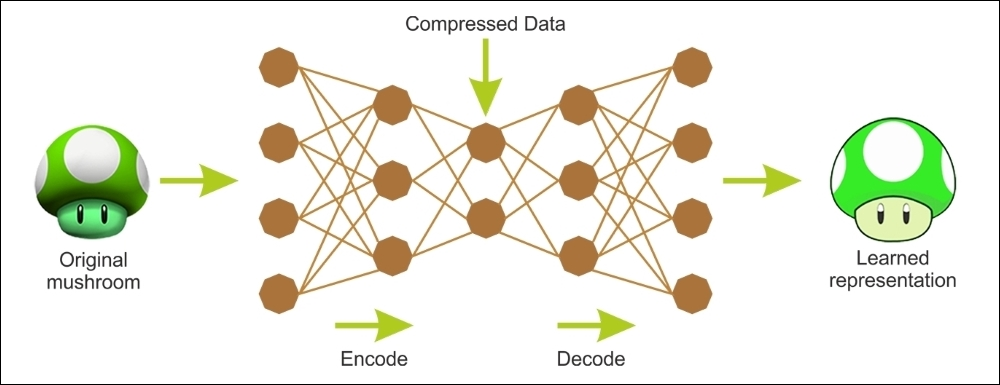Figure 18: Encoding and decoding phases of an autoencoder.

As an unsupervised neural network, the main characteristic of an autoencoder is its symmetrical structure. An autoencoder has two components: an encoder that converts the input to an internal representation, followed by a decoder that converts the internal representation to the output.

In other words, an autoencoder can be seen as a combination of an encoder, where we encode some input into a code, and a decoder, where we decode/reconstruct the code back to its original input as the output. Thus, an MLP typically has the same architecture as an autoencoder, except that the number of neurons in the output layer must be equal to the number of inputs.

As mentioned previously, there is more than one way to train an autoencoder. The first way is to train the whole layer at once, similar to MLP. However, instead of using some labeled output when calculating the cost function, as in supervised learning, we use the input itself. Therefore, the cost function shows the difference between the actual input and the reconstructed input.

### Recurrent Neural Networks (RNNs)

The fundamental feature of an RNN is that the network contains at least one feedback connection, so the activations can flow around in a loop. It enables the networks to do temporal processing and learn sequences, for example performing sequence recognition/reproduction or temporal association/prediction.

RNN architectures can have many different forms. One common type consists of a standard MLP plus added loops. These can exploit the powerful non-linear mapping capabilities of the MLP, and have some form of memory. Others have more uniform structures, potentially with every neuron connected to all the others, and may have stochastic activation functions: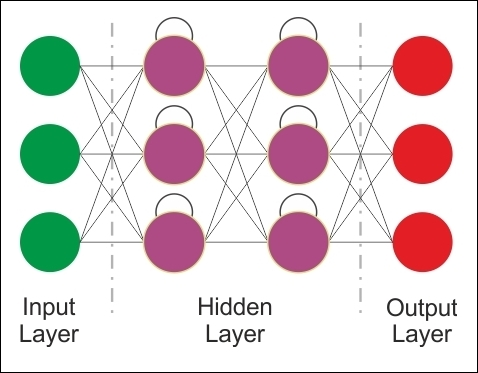Figure 19: RNN architecture.

For simple architectures and deterministic activation functions, learning can be achieved using similar GD procedures to those leading to the backpropagation algorithm for feedforward networks.

The preceding image looks at a few of the most important types and features of RNNs. RNNs are designed to utilize sequential information of input data with cyclic connections among building blocks such as perceptrons, Long Short-term memory units (LSTMs), or Gated Recurrent units (GRUs). The latter two are used to remove the drawbacks of regular RNNs, such as the gradient vanishing/exploding problem and long-short term dependency. We will look at these architectures in later chapters.

### Emergent architectures

Many other emergent DL architectures have been suggested, such as Deep SpatioTemporal Neural Networks (DST-NNs), Multi-Dimensional Recurrent Neural Networks (MD-RNNs), and Convolutional AutoEncoders (CAEs).

Nevertheless, people are talking about and using other emerging networks, such as CapsNets (an improved version of a CNN, designed to remove the drawbacks of regular CNNs), Factorization Machines for personalization, and Deep Reinforcement Learning.

## Deep learning frameworks

In this section, we present some of the most popular DL frameworks. In short, almost all of the libraries provide the possibility of using the graphics processor to speed up the learning process, are released under an open license, and are the result of university research groups.

TensorFlow is mathematical software, and an open source software library, written in Python and C++ for machine intelligence. The Google Brain Team developed it in 2011, and it can be used to help us analyze data, to predict an effective business outcome. Once you have constructed your neural network model, after the necessary feature engineering, you can simply perform the training interactively using plotting or TensorBoard.

The main features offered by the latest release of TensorFlow are faster computing, flexibility, portability, easy debugging, a unified API, transparent use of GPU computing, easy use and extensibility. Other benefits include the fact that it is widely used, supported, and is production-ready at scale.

Keras is a deep-learning library that sits atop TensorFlow and Theano, providing an intuitive API, which is inspired by Torch (perhaps the best Python API in existence). Deeplearning4j relies on Keras as its Python API and imports models from Keras, and through Keras, from Theano and TensorFlow.

François Chollet, a software engineer at Google, created Keras. It runs seamlessly on CPU and GPU. This allows for easy and fast prototyping through user friendliness, modularity, and extensibility. Keras is probably one of the fastest growing frameworks, because it is too easy to construct NN layers. Therefore, Keras is likely to become the standard Python API for NNs.

Theano is probably the most widespread library. Theano is written in Python, which is one of the most widely used languages in the field of ML (Python is also used in TensorFlow). Moreover, Theano allows the use of GPU, which is 24x faster than a single CPU. Theano lets you efficiently define, optimize, and evaluate complex mathematical expressions, such as multidimensional arrays. Unfortunately, Yoshua Bengio announced on 28th September 2017, that development on Theano would cease. That means Theano is effectively dead.

Neon is a Python-based deep learning framework developed by Nirvana. Neon has a syntax similar to Theano's high-level framework (for example, Keras). Currently, Neon is considered the fastest tool for GPU-based implementation, especially for CNN. Although it's CPU-based implementation is relatively worse than most other libraries.

Torch is a vast ecosystem for ML that offers a large number of algorithms and functions, including for DL and for processing various types of multimedia data, with a particular focus on parallel computing. It provides an excellent interface for the C language and has a large community of users. Torch is a library that extends the scripting language Lua and is intended to provide a flexible environment for designing and training ML systems. Torch is a self-contained and highly portable framework on various platforms (Windows, Mac, Linux, and Android) and scripts can run on these platforms without modification. Torch provides many uses for different applications.

Caffe, developed primarily by Berkeley Vision and Learning Center (BVLC), is a framework designed to stand out because of its expression, speed, and modularity. Its unique architecture encourages application and innovation, by allowing an easier transition from CPU to GPU calculations. The large community of users means that considerable development has occurred recently. It is written in Python, but the installation process can be long, due to the numerous support libraries it has to compile.

MXNet is a DL framework that supports many languages, such as R, Python, C++, and Julia. This is helpful because if you know any of these languages, you will not need to step out of your comfort zone at all to train your DL models. Its backend is written in C++ and CUDA and it is able to manage its own memory in a similar way to Theano.

MXNet is also popular because it scales very well and can work with multiple GPUs and computers, which makes it very useful for enterprise. This is why Amazon has made MXNet its reference library for DL. In November 2017, AWS announced the availability of ONNX-MXNet, which is an open source Python package used to import Open Neural Network Exchange (ONNX) DL models into Apache MXNet.

The Microsoft Cognitive Toolkit (CNTK) is a unified DL toolkit from Microsoft Research that makes it easy to train and combine popular model types across multiple GPUs and servers. CNTK implements highly efficient CNN and RNN training for speech, image, and text data. It supports cuDNN v5.1 for GPU acceleration. CNTK also supports Python, C++, C#, and command-line interface.

Here is a table summarizing these frameworks:

Framework

Supported programming languages

Training materials community

CNN modeling capability

RNN modeling capability

Usability

Multi-GPU support

Theano

Python, C++

++

Ample CNN tutorials and prebuilt models

Ample RNN tutorials and prebuilt models

Modular architecture

No

Neon

Python,

+

Fastest tools for CNN

Minimal resources

Modular architecture

No

Torch

Lua, Python

+

Minimal resources

Ample RNN tutorials and prebuilt models

Modular architecture

Yes

Caffe

C++

++

Ample CNN tutorials and prebuilt models

Minimal resources

Creating layers

takes time

Yes

MXNet

R, Python, Julia, Scala

++

Ample CNN tutorials and prebuilt models

Minimal resources

Modular architecture

Yes

CNTK

C++

+

Ample CNN tutorials and prebuilt models

Ample RNN tutorials and prebuilt models

Modular architecture

Yes

TensorFlow

Python, C++

+++

Ample RNN tutorials and prebuilt models

Ample RNN tutorials and prebuilt models

Modular architecture

Yes

DeepLearning4j

Java, Scala

+++

Ample RNN tutorials and prebuilt models

Ample RNN tutorials and prebuilt models

Modular architecture

Yes

Keras

Python

+++

Ample RNN tutorials and prebuilt models

Ample RNN tutorials and prebuilt models

Modular architecture

Yes

Apart from the preceding libraries, there are some recent initiatives for DL on the cloud. The idea is to bring DL capability to big data, with billions of data points and high dimensional data. For example, Amazon Web Services (AWS), Microsoft Azure, Google Cloud Platform and NVIDIA GPU Cloud (NGC) all offer machine and deep learning services (http://searchbusinessanalytics.techtarget.com/feature/Machine-learning-platforms-comparison-Amazon-Azure-Google-IBM) that are native to their public clouds.

In October 2017, AWS released Deep Learning AMIs (Amazon Machine Images) for Amazon Elastic Compute Cloud (EC2) P3 Instances. These AMIs come pre-installed with deep learning frameworks, such as TensorFlow, Gluon and Apache MXNet, that are optimized for the NVIDIA Volta V100 GPUs within Amazon EC2 P3 instances. The deep learning service currently offers three types of AMIs: Conda AMI, Base AMI and AMI with Source Code.

The Microsoft Cognitive Toolkit is Azure's open source, deep learning service. Similar to AWS' offering, it focuses on tools that can help developers build and deploy deep learning applications. The toolkit is installed in Python 2.7, in the root environment. Azure also provides a model gallery (https://www.microsoft.com/en-us/cognitive-toolkit/features/model-gallery/) that includes resources, such as code samples, to help enterprises get started with the service.

On the other hand, NGC empowers AI scientists and researchers with GPU-accelerated containers (see https://www.nvidia.com/en-us/data-center/gpu-cloud-computing/). The NGC features containerized deep learning frameworks such as TensorFlow, PyTorch, and MXNet that are tuned, tested, and certified by NVIDIA to run on the latest NVIDIA GPUs on participating cloud service providers. Nevertheless, there are also third-party services available through their respective marketplaces.

## Summary

In this chapter, we introduced some of the fundamental themes of DL. DL consists of a set of methods that allow an ML system to obtain a hierarchical representation of data on multiple levels. This is achieved by combining simple units, each of which transforms the representation at its own level, starting from the input level, in a representation at a higher and abstraction level.

Recently, these techniques have provided results that have never been seen before in many applications, such as image recognition and speech recognition. One of the main reasons for the spread of these techniques has been the development of GPU architectures that considerably reduce the training time of DNNs.

There are different DNN architectures, each of which has been developed for a specific problem. We will talk more about these architectures in later chapters and show examples of applications created with the TensorFlow framework. This chapter ended with a brief overview of the most important DL frameworks.

In the next chapter, we begin our journey into DL, introducing the TensorFlow software library. We will describe the main features of TensorFlow and see how to install it and set up our first working remarketing dataset.

• ##### Giancarlo Zaccone

Giancarlo Zaccone has over fifteen years' experience of managing research projects in the scientific and industrial domains. He is a software and systems engineer at the European Space Agency (ESTEC), where he mainly deals with the cybersecurity of satellite navigation systems. Giancarlo holds a master's degree in physics and an advanced master's degree in scientific computing. Giancarlo has already authored the following titles, available from Packt: Python Parallel Programming Cookbook (First Edition), Getting Started with TensorFlow, Deep Learning with TensorFlow (First Edition), and Deep Learning with TensorFlow (Second Edition).

Browse publications by this author
• ##### Md. Rezaul Karim

Md. Rezaul Karim is a researcher, author, and data science enthusiast with a strong computer science background, coupled with 10 years of research and development experience in machine learning, deep learning, and data mining algorithms to solve emerging bioinformatics research problems by making them explainable. He is passionate about applied machine learning, knowledge graphs, and explainable artificial intelligence (XAI). Currently, he is working as a research scientist at Fraunhofer FIT, Germany. He is also a PhD candidate at RWTH Aachen University, Germany. Before joining FIT, he worked as a researcher at the Insight Centre for Data Analytics, Ireland. Previously, he worked as a lead software engineer at Samsung Electronics, Korea.

Browse publications by this author

### Latest Reviews

(10 reviews total)